## Labels

php (35) javascript (31) phpjavascript (30) jquery (23) html (20) mysql (14) database (9) codeigniter (4) json (4) bar chart (2) calendar (2) column chart (2) framework (2) google maps (2) query (2) tables (2) url (2) dropdown (1)

# rand()The rand() function outputs a random number. there are two optional parameters that you can add to set mi and max for the number.<?phpecho rand();echo '<br>';echo rand(10,20);?>Result:```str_replace()
This function is useful when you want to do a simple match and replace.
This function accepts four parameters,
three required. find, replace, string, count(optional).
<?php
\$string = 'aaa-bbb-ccc';
echo str_replace('-', '*', \$string);
?>
Result:date()
The date() function can have two parameters,
format(required) and timestamp(optional).
<?php
echo date("d-m-Y h:i:s");
?>
Result: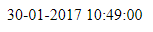strlen()
The strlen function will return the length of a given string.
The spaces and characters are added to the count.<?php
\$stringOne = "Hello PHP";
echo 'stringOne Length = ' . strlen(\$stringOne) . '<br><br>';
\$stringTwo = " Welcome code";
echo 'stringTwo Length = ' . strlen(\$stringTwo);
?>
Result:count()
This function count the number of element in a array.
<?php
\$Alphabets = array("A", "B", "C", "D", "E");
echo 'Count = '.count(\$Alphabets);
?>
Result: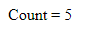array_combine()
This function creates an array by using
one array for keys and another for its values.
<?php
\$fruit_color = array("green", "red", "yellow");
\$fruits_array= array_combine(\$fruit_color, \$fruits);
print_r(\$fruits_array);
?>
Result:array_unique()
You can use this function to removes duplicate values from an array.
<?php
\$input = array("a" => "green", "red", "b" => "green", "blue", "red");
\$result = array_unique(\$input);
print_r(\$result);
?>
Result: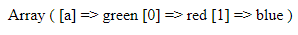print_r()
This function is used to print array variable in a way that’s readable by humans.
<?php
\$fruits = array ("a" => "Banana", "b" => "Apple");
print_r (\$fruits);
?>
Result:strrev()
This function reverses the given string.
<?php
echo strrev("Hello PHP");
?>
Result: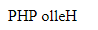trim()
This function removes characters, space from the string form both the ends.
<?php
\$str = "Hello PHPJavaScript";
echo \$str . "<br>";
echo trim(\$str,"Ht");
?>
Result: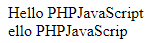ucfirst()
This function Converts the first character to uppercase.
<?php
echo ucfirst("hello php!");
?>
Result:For all PHP built in functions, go to  PHP.NET
Please do share and fallow our blog for more intresting content.

```